We aim here at modeling the spread of the COVID-19 epidemics in Slovenia over time using the data on the infected individuals in Slovenia. For modeling the temporal dynamics of the population of infected individuals, we use a Kermack-McKendrick variant of the SIR epidemiological model. We fit the model parameters to the data using the modeling tool ProBMoT. In turn, we use the model to simulate and predict the dynamics of the infected population in the future. From the predicted dynamics, we can infer the expected time point of the infection peak, the number of infected individuals at the peak and the expected time point of the epidemics demise.

# The model

The Kermack-McKendrick variant of the SIR model of the temporal spread of the infectuous disease in a closed, homogeneous population of individuals. It comprises three differential equations modeling the change of the three variables S, I and R, corresponding to the size of the populations of susceptible (S), infected (I) and recovered (R) individuals: $\frac{d S}{d t} = - \beta S I \\ \frac{d I}{d t} = \beta S I - \gamma I \\ \frac{d R}{d t} = \gamma I .$ The two constant parameters of $$\beta$$ and $$\gamma$$ represent the rates of infection and recovery, respectively.

Given the population sizes at the initial time point $$t_0$$, $$S(t_0)$$, $$I(t_0)$$ and $$R(t_0)$$, along with the values of $$\beta$$ and $$\gamma$$, we can simulate the dynamical change of the three populations through time. At any simulation time point $$t$$, the sum $$S(t) + I(t) + R(t)$$ is constant and equals the size of the whole population.

# Fitting the model to the data

The data used for fitting the model comprises the time-series corresponding to the dynamic change of the number of infected individuals in Slovenia from 14th of March to the current date obtained from the Covid-19 sledilnik Website. After the initial experiments on these dates, we realized that the trend of the time-series of the number of infected individuals changed notably on 14th of March, when Slovene government revised the testing policy. After the initial set of preliminary experiment, we decided to trim the initial time-series, so they start on 14th of March, when the revised testing policy has been implemented.

To obtain the values of the model parameters $$\beta$$ and $$\gamma$$, we fit the model agains the observations of the number of infected individuals in Slovenia. To this end, we use the ProBMoT tool for data- and knowledge-driven modeling of dynmical systems. ProBMoT formulates the fitting of the model parameters to observed data as a task of numerical optimization, where the objective is to minimize the discrepancy between the observations $$I$$ and simulated values $$\hat{I}$$ of the size of the infected population. The discrepancy is measured using the standard root-mean-square-error (RMSE):

${RMSE} = \sqrt{ \frac{1}{n} \sum _{t = t_0} ^{t_{n-1}} (I(t) - \hat{I}(t))^2},$ where $$n$$ is the number of observations at the time points $$t_0, t_1, \ldots t_{n-1}$$. Note that $$RMSE$$ is measured using the simulated behavior of the model $$\hat{y}$$: ProBMoT simulates the SIR model using the CVODE solver of ordinary differential equations as implemented in the Sundials suite.

Beside the model parameters $$\beta$$ and $$\gamma$$, we also treat the three population sizes at the initial time point $$t_0$$ as free parameters to be fitted against the observations. Thus, the parameter fitting of the SIR model leads to a five-dimensional numerical optimization problem. To solve it, ProBMoT employs the Differential Evolution algorithm with standard settings and a population size of 100. The obtained solution of the optimization problem assigns values to the fitted parameters that lead to the minimal RMSE discrepancy between the observations and the simulations.

# Simulating the model

Once we fit the values of the initial population sizes and the two rate parameters, we can simulate the model beyond the last observation point $$t_{n-1}$$ and therefore obtain prediction of the infection dynamics.

However, the observations are severely limited. First, we observe only few initial points at the very beginning of the epidemics with the (rapidly) growing size of the population of infected individuals. Second, the observations are based on a very limited sample of individuals that have been tested. On such a small set of observations, we face a high risk of overfitting. While the bias component of the fitting error can be kept at bay by assuming the SIR model, the variance of the parameter estimates is out of control.

To account for the high variance, of the parameter estimates, we perform a bootstrap simulation of the model as opposed to a single model simulation with the optimally fitted parameter values. The bootstrap simulation procedure samples the parameter values in two ways. First, we sample all the parameter values considered by the Differential Evolution algorithm during the optimization: given the algorithm settings, 2,000 evaluations are performed. We constrain the sampling to those parameter values that lead to RMSE, which is within a 25% margin of the RMSE of the optimal parameter values, i.e., lies within the interval $$[RMSE_{opt}, RMSE_{opt} \cdot 1.25]$$, where $${RMSE}_{opt}$$ is the error obtained with the optimal parameter values.

Second, given this initial set of parameter values, we sample their neighborhoods from the Gaussian distribution $$N(p_0, \sigma_p)$$, where $$p_0$$ and $$\sigma_p$$ are estimated from the ProBMoT evaluations selected in the first phase. Again, we constrain the sampling to those parameter values that lead to RMSE, which is less than a half of the RMSE of the simplest model that predicts the mean of the observed values.

For each sample of parameter values, we simulate the model for a period of time from the 14th of March 2020 to the 1st of July 2020. The simulations are aggregated using minimal and maximal simulated values at each time point leading to the graph in Figure 1.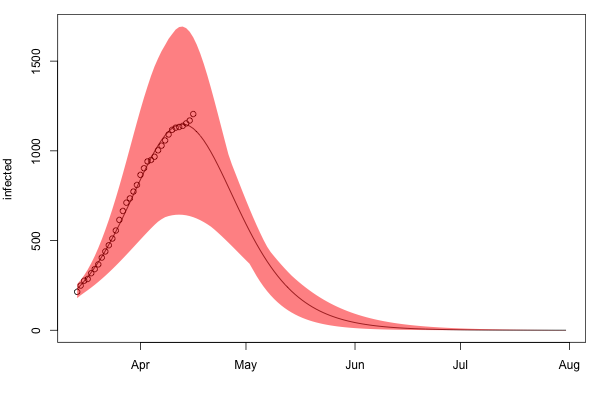Figure 1: The bootstrap simulation (red polygon) of the SIR model fitted to the observations of the number of infected individuals in Slovenia from 14th of March to 16th of April 2020 (black circles). The solid black line within the polygon depicts the simulation of the model with optimal parameter values (lowest RMSE).

# Analysis of the simulations

From the simulations, we would like to predict two important time points of the epidemics. The first is the time position of the extreme size of the infected population, which we refer to as peak. The second is the time point of the epidemics demise, i.e., a time point after the peak, when the infected population size diminishes to at most 10 infected individuals.

When considering the peak, both, the magnitude and the time point of the peak are of interest. The series of figures 2a-k and 3a-k (later in the Appendix) depict the distribution of the predicted peak time points and the predicted peak magnitudes in the bootstraped simulations of the updated models. After the first five updates of the model, we put the predictions into perspective in Figures 2 and 3.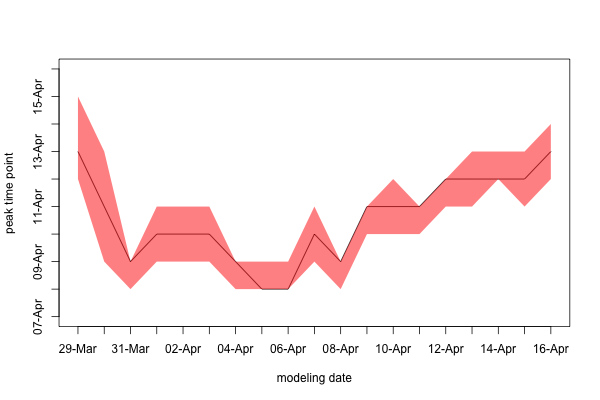Figure 2: Temporal change of the predicted peak time point with the model updates: the black line corresponds to the median predictions, while the red polygon is based on the first and the thirth quartile of the predictions.

MMajority of the bootstraped simulations predict the median time point of the peak to be between the 8th and 13th of April. The model updates in the beginning of April stabilized the prediction of the median value on 10th of April, than slid down towards the 8th of April and got back to the 13th of April.

This still might be considered an optimistic estimate since the observed size of the infected population is unrealistically small due to the policy of limited, non-systematic testing for Covid-19 in Slovenia. Note also that the model learned from data staring at 4th of March lead to even more optimistic estimates. In particular, due to the change of the testing policy in mid March, the observed dynamics of the number of infected individuals has slowed down, which leads to smaller estimates of the infection rate, thus leading to early peak estimate. Which was the reason we have trimmed the training data of the model so it starts on 14th of March, the date when the revised testing policy was introduced.

On the other hand, note that the number of recovered individuals in Slovenia is reported to be constant (16) for more than 10 days in a row. This means, that the status of some of the infected individuals is not being updated, once an individual is registered as infected, it is not clear whether and when his/her status is being updated to recovered. This means, that, despite the obvious inconsistency with the empirical data, the model might have correctly predicted the peak of the number of infected individuals to be between 9th and 12th of April.

Data update on 14th of April: the data on number of recovered individuals was revised for the whole period from 4th to 14th of April, so the numebr of recovered individuals in the whole period is 26, as oposed to 16, the number before the update. Despite this abrupt data update, the model predictions are still stable: the median preicted time point of the peak is still between 12th and 13th of April.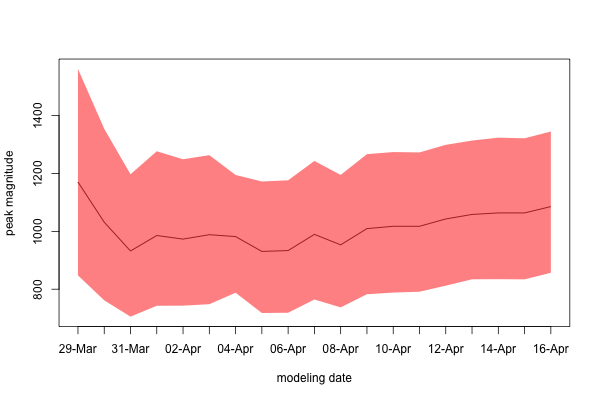Figure 3: Temporal change of the predicted peak magnitude with the model updates: the black line corresponds to the median predictions, while the red polygon is based on the first and the thirth quartile of the predictions.

With the model updates, the median predictions of the magnitude of the peaks vary between 900 and 1,200. After the initial decrease observed in the March updates, the median prediction is quite stable and varies between 950 and 1,100, with a slight increase to more than 1,000 towards the end of the update period: current median prediction being 1,086. Note that this is definitely optimistic, given that the predictions are models learned from data based on relatively modest rates of testing in Slovenia. Note also, that the current median prediction is slightly smaller than the observed number of the infected individuals.

Note again, that, despite the obvious inconsistency with the empirical data, the model might have still correctly predicted the magnitude of the magnitude of the peak of the number of infected individuals. Despite the abrupt data update and revision on 14th of April, the prediction of the updated model remain stable showing a slight increasing trend in the most recent updates.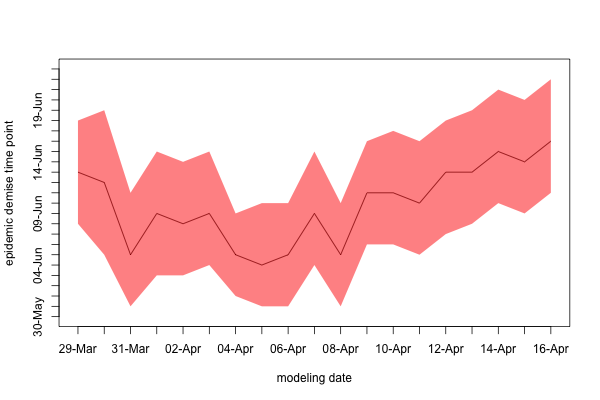Figure 4: Temporal change of the predicted time point of the epidemic demise with the model updates.

Figure 4 depicts the prediction of the time point of the epidemics demise in Slovenia. Majority of the bootstraped simulations predict that the epidemic demise is to be expected by mid June with a median varying between 4th and 13th of June. The prediction trend at the beginning of April pointed towards earlier dates in the first half of June with an increase towards the 16th of June with the most recent model updates. For the reasons similar to the ones outlined above, this estimate tend to be optimistically premature as well.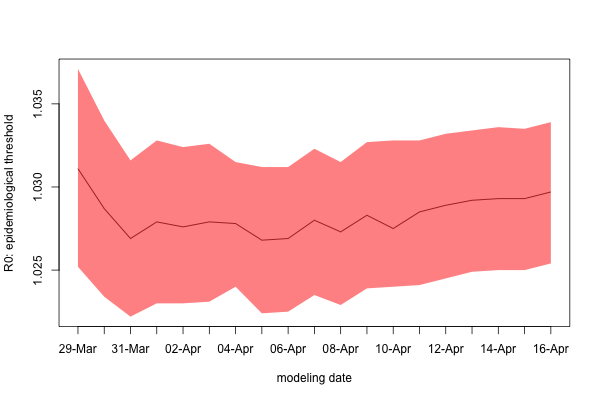Figure 5: Temporal change of the epidemiological threshold $$R_0$$ with the model updates: the black line corresponds to the median vlues, while the red polygon is based on the first and the thirth quartile.

Finally, Figure 5 depicts the change of the epidemiological threshold $$R_0$$, i.e., the number of individuals infected by contact with a single infected person before her/his recovery. The median value of $$R_0$$ monotonlically decreased with the early model updates, which is a plausible consequence of the introduction of the social distancing measures. With the later updates the value stabilized at values lower than 1.03, the medeain value of 1.0297 being predicted with the last model update.

# Appendix

## Simulation of the previous model updates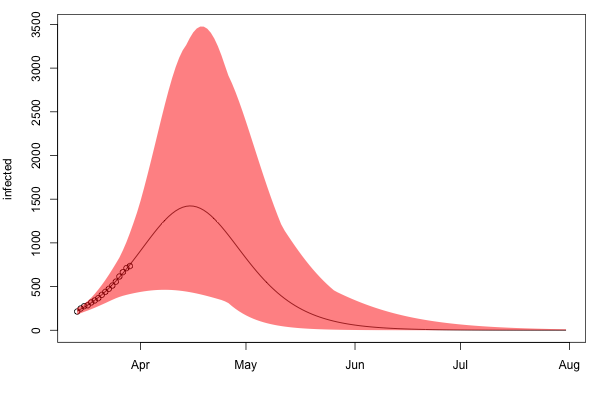Figure 1a: Bootstrap simulation of the model updated with the number of infected individuals in Slovenia up to 29th of March 2020.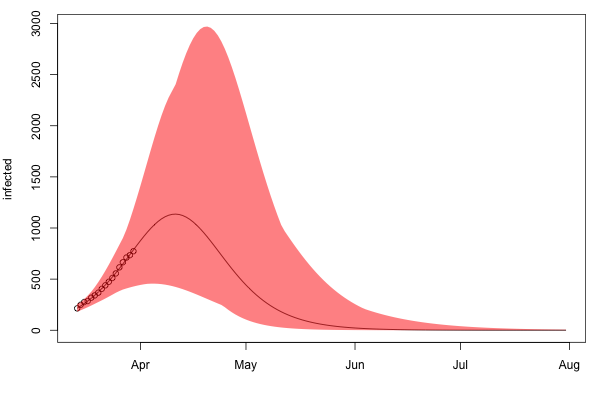Figure 1b: Bootstrap simulation of the model updated with the number of infected individuals in Slovenia up to 30th of March 2020.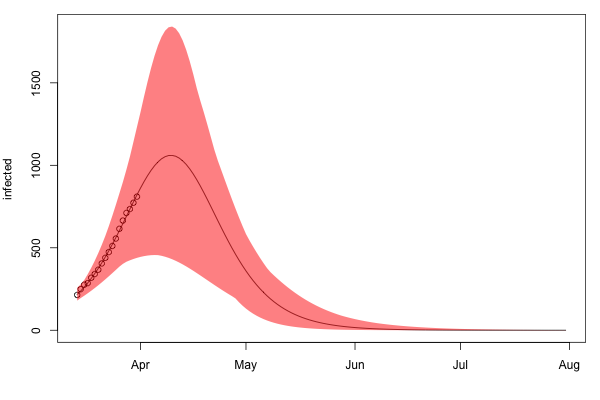Figure 1c: Bootstrap simulation of the model updated with the number of infected individuals in Slovenia up to 31st of March 2020.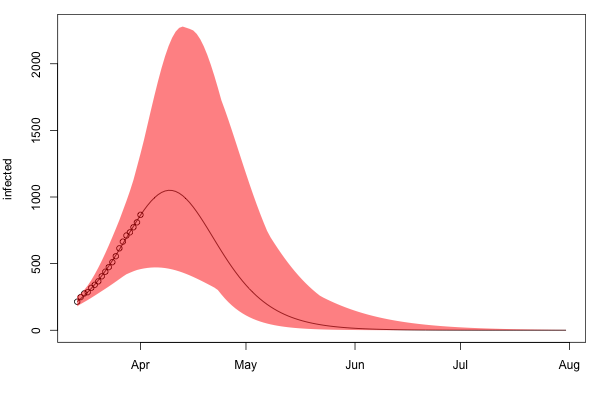Figure 1d: Bootstrap simulation of the model updated with the number of infected individuals in Slovenia up to 1st of April 2020.